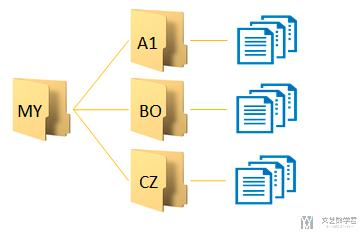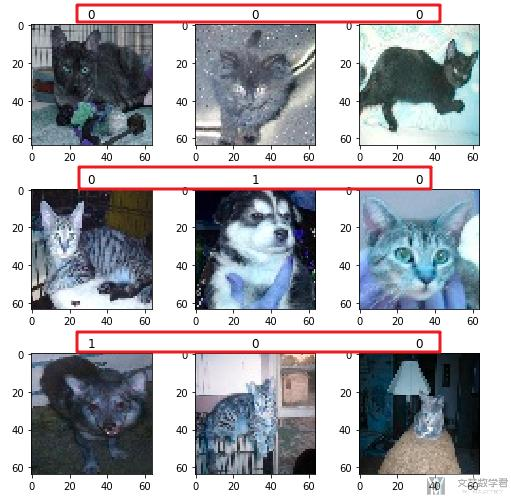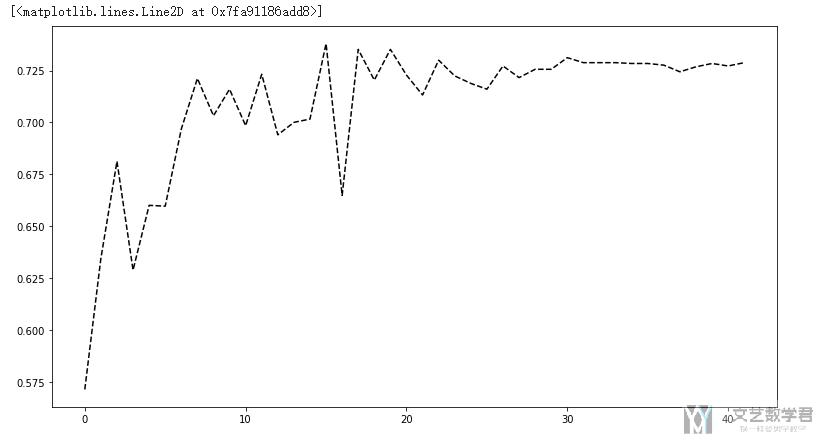# 将图像数据存储为npy文件

• A+

## 本地数据集保存为npy格式

### 准备工作### 开始转换, 图片转换为npy格式

• t10k-labels-idx1, 测试集标签
• t10k-images-idx3, 测试集数据
• train-labels-idx1, 训练集标签
• train-images-idx3, 训练集数据

• 首先遍历根目录下所有子目录和其中的文件
• 将内容与label一起保存在numpy中
• 打乱顺序
• 输出四个文件.

1. def image_label(imageLabel, label2idx, i):
2.     """返回图片的label
3.     """
4.     if imageLabel not in label2idx:
5.         label2idx[imageLabel]=i
6.         i = i + 1
7.     # 返回的是字典类型
8.     return label2idx, i

1. def image2npy(dir_path='./dogs_cats/', testScale = 0.1):
2.     """生成npy文件
3.     """
4.     i = 0
5.     label2idx = {}
6.     data = []
7.     for (root, dirs, files) in os.walk(dir_path):
8.         for Ufile in tqdm(files):
9.             # Ufile是文件名
10.             img_path = os.path.join(root, Ufile) # 文件的所在路径
11.             File = root.split('/')[-1] # 文件所在文件夹的名字, 也就是label
12.             # 读取image和label数据
14.             label2idx, i = image_label(File, label2idx, i)
15.             label = label2idx[File]
16.             # 存储image和label数据
17.             data.append([np.array(img_data), label])
18.     random.shuffle(data) # 随机打乱,直接打乱data
19.     # 训练集和测试集的划分
20.     testNum = int(len(data)*testScale)
21.     train_data = data[:-1*testNum] # 训练集
22.     test_data = data[-1*testNum:] # 测试集
23.     # 测试集的输入输出和训练集的输入输出
24.     X_train = np.array([i for i in train_data]) # 训练集特征
25.     y_train = np.array([i for i in train_data]) # 训练集标签
26.     X_test = np.array([i for i in test_data]) # 测试集特征
27.     y_test = np.array([i for i in test_data]) # 测试集标签
28.     print(len(X_train), len(y_train), len(X_test), len(y_test))
29.     # 保存文件
30.     np.save('train-images-idx3.npy', X_train)
31.     np.save('train-labels-idx1.npy', y_train)
32.     np.save('t10k-images-idx3.npy', X_test)
33.     np.save('t10k-labels-idx1.npy', y_test)
34.     return label2idx

2. img_data = cv2.resize(img_data, (64, 64)) # 图片处理成统一大小

### 结果检查

1. # 随机检查label与图片是否可以对应上
2. # - 从train中抽取9个image和9个label
3. image_no = np.random.randint(0,3602, size=9) # 随机挑选9个数字
6. fig, axes = plt.subplots(nrows=3, ncols=3,figsize=(7,7))
7. for i in range(3):
8.     for j in range(3):
9.         axes[i][j].imshow(train_images[image_no[i*3+j]])
10.         axes[i][j].set_title(train_labels[image_no[i*3+j]])
11. plt.tight_layout()## 使用npy结合Colaboratory进行训练

2. # ---------
3. # 转换为tensor
4. # ---------
5. X_train = torch.from_numpy(train_images.reshape(-1, 3, 64, 64)).float() # 输入 x 张量
6. X_test = torch.from_numpy(test_images.reshape(-1, 3, 64, 64)).float()
7. Y_train = torch.from_numpy(train_labels).long() # 输入 y 张量
8. Y_test = torch.from_numpy(test_labels).long()
9. print(X_train.shape, Y_train.shape)
10. # ---------------
12. # ---------------
13. MINIBATCH_SIZE = 200
14. trainDataset = torch.utils.data.TensorDataset(X_train, Y_train) # 合并训练数据和目标数据
16.     dataset=trainDataset,
17.     batch_size=MINIBATCH_SIZE,
18.     shuffle=True,
19.     num_workers=1           # set multi-work num read data
20. )
21. testDataset = torch.utils.data.TensorDataset(X_test, Y_test) # 数据路径
23.     dataset=testDataset,
24.     batch_size=MINIBATCH_SIZE, # 批量大小
25.     shuffle=True# 乱序
26.     num_workers=1 # 多进程
27. )1. Time 2020-04-05 22:20:18.217865, Epoch [41/42], Step [29/113], loss:0.0001
2. Training Accuracy: 100.0, Training Rate: 0.00022876792454961005
3. Time 2020-04-05 22:20:28.220307, Epoch [41/42], Step [59/113], loss:0.0002
4. Training Accuracy: 100.0, Training Rate: 0.00022876792454961005
5. Time 2020-04-05 22:20:38.219144, Epoch [41/42], Step [89/113], loss:0.0001
6. Training Accuracy: 100.0, Training Rate: 0.00022876792454961005
7. Test Accuracy of the model on the 10000 test images: 72.88 %

## 参考资料

Why you should start using .npy file more often…

How to Save a NumPy Array to File for Machine Learning

Data preparation for deep learning: images to a .npy file

• 微信公众号
• 关注微信公众号
•• QQ群
• 我们的QQ群号
•